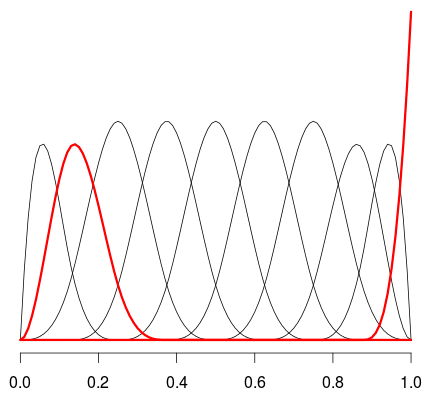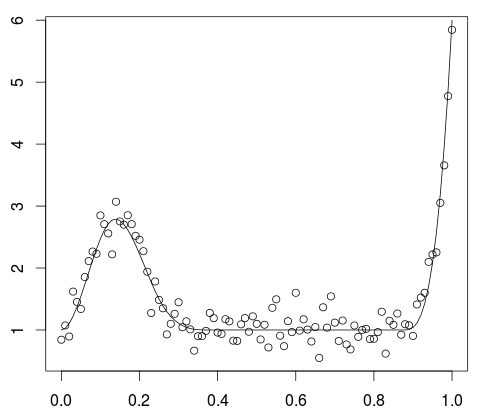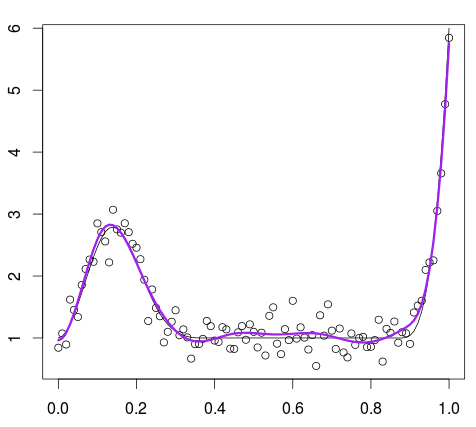Regression with Splines: Should we care about Non-Significant Components?

January 4, 2016
By

(This article was first published on Freakonometrics » R-english, and kindly contributed to R-bloggers)

Following the course of this morning, I got a very interesting question from a student of mine. The question was about having non-significant components in a splineregression.  Should we consider a model with a small number of knots and all components significant, or one with a (much) larger number of knots, and a lot of knots non-significant?

My initial intuition was to prefer the second alternative, like in autoregressive models in R. When we fit an AR(6) model, it’s not really a big deal if most coefficients are not significant (but the last one). It’s won’t affect much the forecast. So here, it might be the same. With a larger number of knots, we should be able to capture small bumps that we’ll never capture with a smaller number.

Here is what a have with a small number of knots, and cubic splinesand with a larger number of knotsIn order to understand what’s going on, consider a simple model, with the two splines above, in red

> set.seed(1)
> library(splines)
> x=seq(0,1,by=.01)
> v=bs(x,10)
> x2=v[,2]
> x10=v[,10]
> set.seed(1)
> y=1+3*x2+5*x10+rnorm(length(x))/4
> y_test=1+3*x2+5*x10+rnorm(length(x))/4

Note that here I have generated two sets of data, one to train a model, and one to test it.  Here, the data looks like that

> plot(x,y)It is based on two splines,

> lines(df\$x,1+3*x2+5*x10)If we use a spline model with 10 degrees of freedom, we get

> df=data.frame(x,y)
> reg=lm(y~bs(x,10),data=df)
> summary(reg)

Coefficients:
Estimate Std. Er t value Pr(>|t|)
(Intercept)  0.91671 0.17068   5.371 6.08e-07 ***
bs(x, 10)1   0.20485 0.32696   0.627    0.533
bs(x, 10)2   3.15593 0.22534  14.005  < 2e-16 ***
bs(x, 10)3   0.04847 0.25075   0.193    0.847
bs(x, 10)4   0.09373 0.21597   0.434    0.665
bs(x, 10)5   0.11624 0.22939   0.507    0.614
bs(x, 10)6   0.24829 0.22293   1.114    0.268
bs(x, 10)7  -0.06825 0.23498  -0.290    0.772
bs(x, 10)8   0.19633 0.26241   0.748    0.456
bs(x, 10)9   0.27557 0.26976   1.022    0.310
bs(x, 10)10  4.78134 0.24116  19.826  < 2e-16 ***

which makes sense, from what we have generated. Indeed, most of the components are not significant, but the second and the tenth. We can actually test that all those components are null (at the same time)

> A=matrix(0,8,11)
> colnames(A)=names(coefficients(reg))
> A[1,2]=A[2,4]=A[3,5]=A[4,6]=A[5,7]=
+ A[6,8]=A[7,9]=A[8,10]=1
> b=rep(0,8)
> linearHypothesis(reg, A,b)
Linear hypothesis test

Hypothesis:
bs(x, 10)1 = 0
bs(x, 10)3 = 0
bs(x, 10)4 = 0
bs(x, 10)5 = 0
bs(x, 10)6 = 0
bs(x, 10)7 = 0
bs(x, 10)8 = 0
bs(x, 10)9 = 0

Model 1: restricted model
Model 2: y ~ bs(x, 10)

Res.Df    RSS Df Sum of Sq      F Pr(>F)
1     98 4.8766
2     90 4.6196  8   0.25701 0.6259  0.754

and yes, those coefficients are not significant.

> yp10=predict(reg)
> lines(df\$x,yp10,col="red")The red curve is not far away from the black one (the simulated model, when the noise is removed).

Now, if we compare with a spline regression with three degrees of freedom

> reg=lm(y~bs(x),data=df)
> yp3=predict(reg)
> lines(df\$x,yp3,col="blue",lwd=3)
> summary(reg)

Coefficients:
Estimate Std. Err t value Pr(>|t|)
(Intercept)   1.5381  0.2188   7.029 2.91e-10 ***
bs(x)1        2.2276  0.6348   3.509 0.000683 ***
bs(x)2       -4.4788  0.4089 -10.952  < 2e-16 ***
bs(x)3        1.9936  0.3434   5.805 8.12e-08 ***

This time, all components are significant, but the quality of fit is rather poor, isn’t it?What is the best thing we can do? Let us fit different models. Say consider 8 degrees of freedom, but keep only 1, or 2, or 3, etc components. Then get a prediction on the test-dataset we have, and see which model has the smallest variance,

> me=1e9
> ERR=matrix(NA,15,15)
> for(k in 4:15){
+ bsX=bs(df\$x,k)
+ dfX=data.frame(y=df\$y,bsX)
+ reg=lm(y~.,data=dfX)
+ library(leaps)
+ W=leaps( bsX , df\$y , method="Cp" ,
+ nbest=1)\$which
+ for(i in 1:k){
+ dfXi=data.frame(y=df\$y,bsX[W[i,]])
+ reg=lm(y~.,data=dfXi)
+ dfXi_s=data.frame(y=y_test,bsX[,W[i,]])
+ names(dfXi_s)=c("y",
+ names(coefficients(reg))[-1])
+ erreur=dfXi_s\$y-predict(reg,newdata=dfXi_s)
+ ERR[k,i]=sum(erreur^2)
+ if(ERR[k,i]<=me){LOC=c(k,i);me=ERR[k,i]}
+ }}

The best model is when we keep the ten best components, out of 11 degrees of freedom

> LOC
 11 10

More specifically,

> library(leaps)
> bsX=bs(df\$x,LOC)
> W=leaps( bsX , df\$y , method="Cp" ,
+ nbest=1)\$which
> dfXi=data.frame(y=df\$y,bsX[,W[LOC,]])
> reg=lm(y~.,data=dfXi)
> ypopt=predict(reg)
> lines(df\$x,ypopt,col="purple")The best model here is one with more degrees of freedom than the one we used to generate the data,

Actually, if we use 10 degrees of freedom, but keep only the best two components, we get something rather close

> bsX=bs(df\$x,10)
> W=leaps( bsX , df\$y , method="Cp" ,
+ nbest=1)\$which
> dfXi=data.frame(y=df\$y,bsX[,W[2,]])
> reg=lm(y~.,data=dfXi)
> ypopt=predict(reg)
> lines(df\$x,ypopt,col="red",lwd=3)So, it looks like having a lot of non significant components in a spline regression is not a major issue. And reducing the degrees of freedom is clearly a bad option.

R-bloggers.com offers daily e-mail updates about R news and tutorials on topics such as: Data science, Big Data, R jobs, visualization (ggplot2, Boxplots, maps, animation), programming (RStudio, Sweave, LaTeX, SQL, Eclipse, git, hadoop, Web Scraping) statistics (regression, PCA, time series, trading) and more...

If you got this far, why not subscribe for updates from the site? Choose your flavor: e-mail, twitter, RSS, or facebook...